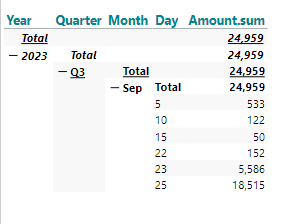# Dynamic Time in Data Discovery with "empty" dates

If you build a cube in data discovery based on just one data source with some transactions, you might encounter a problem with dynamic date filters when they refer to dates not represented in your data.

As an example - we can look at this data source (excel file) with a few transaction: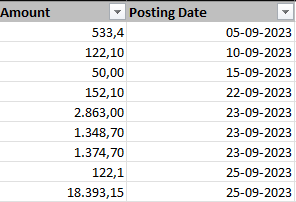If we build a cube based on this data alone, we can make a table like this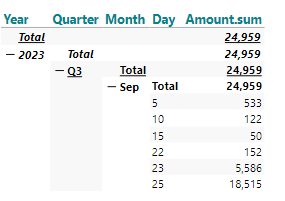But when we apply a filter like month to date (based on the date being 26th of September 2023), we get an error like this: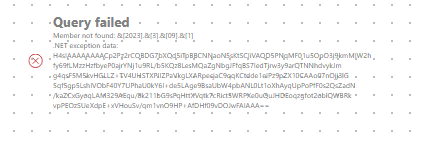The error comes because neither the 1st of September nor the 26th of September are represented in the dataset.

The workaround will be to introduce a dimensions table representing all dates and a fact table representing a value (dummy value) for each date.

Dimension table should look like this: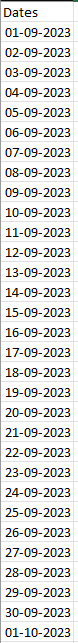And fact table could look like this (almost similar):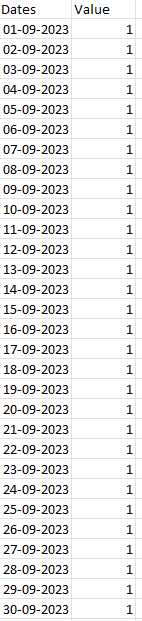Now we can expand our cube to this model: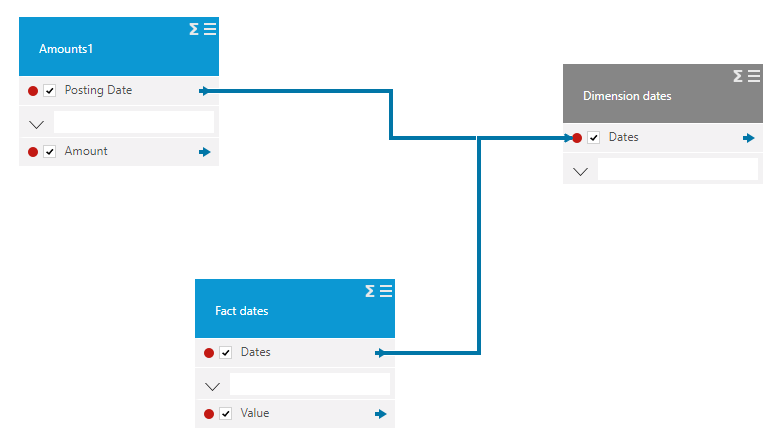Using my new Date from the Dimension Dates table as filter and dimension in the table, we can now apply month to date filter and get this result: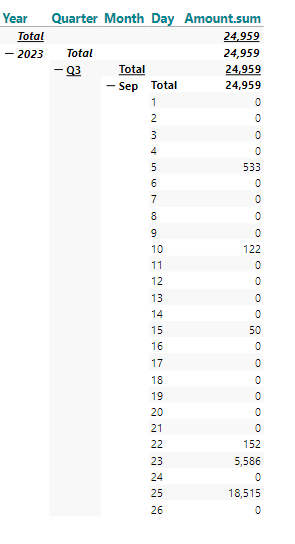Now we just need to apply a visibility agent that hides all rows with value = 0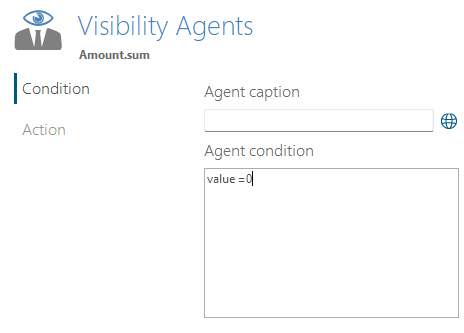And the table is now shown according to the filter: